## 2.12Newtonian ﬂuid

In Sec. 2.6 we introduced forces in a ﬂuid. At the macroscopic scale of a continuum, we characterise a ﬂuid’s response to applied forces through constitutive models.

The Newtonian (or linear viscous) ﬂuid is the most common constitutive model that represents the behaviour of many liquids and gases. It states that a ﬂuid at rest (or uniform velocity) does not sustain shear stress; it can be expressed by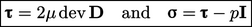(2.41)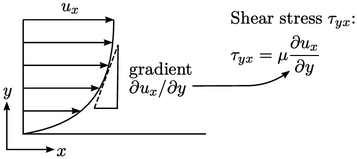The model is a continuum representation9 of Newton’s law of viscosity10 which states that shear stress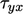is proportional to velocity gradient by the dynamic viscosity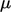.

It is a speciﬁc case of the more general Stokesian ﬂuid, deﬁned as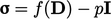, where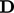is deformation rate, Eq. (2.33 ). The Newtonian model assumes: (1) the ﬂuid is isotropic, i.e. the value ofis independent of the direction in which it is measured; (2) zero bulk viscosity associated with a change in volume o the ﬂuid.

Usingin Eq. (2.41 ) ensures that the shear stress is induced by deformation only. Taking the deviatoric part,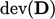, ensures viscous stresses are not generated by volume changes, which are represented by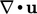.

This is due to the “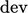” operator subtracting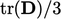from each diagonal component of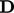, giving a total of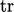() from all 3 diagonal components. From Eq. (2.35 ),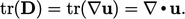When we substitute Eq. (2.41) in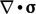in Eq. (2.19 ), the pressure part is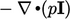. The term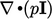is equivalent to gradient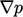.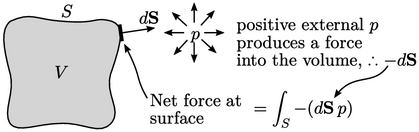Like divergence of stress in Eq. (2.22 ), the gradient of pressure represents the pressure ﬂux across the surface per unit volume as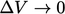, according to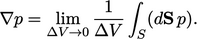(2.42)
Since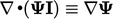holds for any variable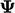, a gradient term is conservative, like divergence, and can be converted to a surface integral under an equivalent Gauss’s Theorem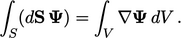(2.43)

9attributed to Adhémar Jean Claude Barré de Saint-Venant, 1843, and George Gabriel Stokes, 1845; derived previously using molecular models by Claude-Louis Navier, Mémoire sur les lois du mouvement des ﬂuides, 1822.
10Isaac Newton, Philosophiae Naturalis Principia Mathematica, 1687.

Notes on CFD: General Principles - 2.12 Newtonian ﬂuid